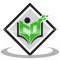## Relation Between Bar and PascalUpdated on 13-Oct-2022 11:19:47
Introduction The bar is often used in order to express the atmospheric pressure. The atmospheric air that is often provided in millibars and the standard pressure is determined as the atmospheric pressure is denoted as 1013.25 mbar 101.325 kPa 1.01325 bars. This principle is used to state the relation between Pascal and bar, where about 14.7 pound/inch2. In this case, bar is determined as the metric unit of pressure and is not the part of SI units or the system of International Units . The bar can also be defined as the metric unit that is equivalent to 100, 000 ... Read More

## RegelationUpdated on 13-Oct-2022 11:19:47
Introduction If the freezing point of water gets lowered after applying a certain amount of pressure on it then this process is determined as regelation. Due to regelation ice gets melted to water at 0°C temperature under pressure and again gets frozen back to ice on the pressure removal. The glacier is considered to be the source of the river, as water in the river comes from glaciers due to the regelation of ice. On snow or ice skating is only possible due to water formation for the regelation. Here water is formed as the pressure increases and serves as ... Read More

## Difference Between Stress and PressureUpdated on 13-Oct-2022 11:19:47
Introduction Stress and pressure are both forces that are often misinterpreted; however, pressure is defined as the physical force applied to an object. In simpler words, pressure is an amount of force that is applied per unit area. Pressure is majorly noticed as the unique property of thermodynamics and serves as a cause for the aspect of stress. On the other hand, stress is referred to as the material property and it occurs due to the result of the pressure exerted. However, both the forces are crucial as they are used in the field of physical science and engineering ... Read More

## Thrust PressureUpdated on 13-Oct-2022 11:19:47
Introduction Thrust is the perpendicular acting force on the surface and pressure is the exerted thrust "per unit of a body". Newtown is the SI unit used for the calculation of the thrust of an object. According to physics, the force which is applied on a surface can regulate the object’s body movement as a perpendicular force on the surface of the following object. Additionally, thrust is the upward force which is applied on the surface of an object. Define Thrust The acting strength that is vertical to the object’s surface is thrust in physics. It is conveyed by 'T'. ... Read More

## SphygmomanometerUpdated on 13-Oct-2022 11:19:47
Introduction Sphygmomanometer, the original blood pressure device is used for measuring the blood pressure. This machine or instrument is used in the medical field and it has its importance. It has different names like blood pressure meter and blood pressure gauge. It includes a measuring unit, an inflatable cuff, and a device to inflate the cuff. It is used for measuring blood pressure and blood pressure stands for force applied to the artery walls. Definition of a sphygmomanometer Sphygmomanometer is an instrument used in the medical field for the measurement of blood pressure. It is also known as ... Read More

## Relation Between Bar And AtmUpdated on 13-Oct-2022 11:19:47
Introduction Pressure is the term that is used in several sections of physics. The term, pressure is given in the form of different units. The Bar and Atm are the two units that help in the representation of pressure. The bar The Atm as the pressure metric unit denote the atmospheric pressure The word bar is derived from the Greek word, baros, which means weight. Relationship between bar and ATM A bar is a metric unit that is used to determine the amount of pressure; however, this is not the part of ... Read More